﻿ Investigation of Oil Reservoir Vibration under the Impact of Earthquake in Proper and Corrosion-Occurred Tanks

### Investigation of Oil Reservoir Vibration under the Impact of Earthquake in Proper and Corrosion-Occu...OPEN ACCESSPEER-REVIEWED

## Investigation of Oil Reservoir Vibration under the Impact of Earthquake in Proper and Corrosion-Occurred Tanks

Afshin Mansouri1,, Babak Aminnejad2

1Department of Civil Engineering, Master of civil Engineering, The University of Roudehen Branch, Tehran, Iran

2Department of Civil Engineering, Faculty of Civil Engineering, The University of Roudehen Branch, Tehran, Iran

### Abstract

Steel cylindrical reservoirs had the highest utilization in the field of oil source storage and petrochemical in the recent years due to their significant importance. These types of reservoirs are conventionally made with steady and floating roofs. Long term erosion agents have destructive impacts on dynamic features of these reservoirs. Results of numerical researches demonstrate that the internal corrosion of reservoir walls as a constant dependent to time, which is made due to the connection of fluids and chemical interactions with the internal wall of reservoir causes the corrosion of inside the reservoir wall and reduction in wall stiffness. In this research dynamic behavior of three models of steel cylindrical reservoirs (with the same height to diameter proportion) containing fluid is modeled using ANSYS software by applying the finite element method. In this modeling, features of a cylindrical reservoir containing 0.9 height of liquid is used which its fluid is considered to be incompressible and viscose. First Modal and Harmonic analyses are used to evaluate the natural frequency and formed mode-shapes in the tank-fluid system. These models are compared and verified with the similar and current experimental formulas. Next by applying corrosion on one of the tanks, dynamic features are evaluated using the software. Then some mass is applied in the place of corrosion to the walls of reservoir with proper boundary conditions in several steps, which in each step, the changes in the added mass are considered using the transient analysis and finally the natural frequency is evaluated. This cycle continued until the frequency of reservoir having added mass has equaled to the frequency of corrosion reservoir which this method is called equivalent mass. In the end, the added mass to the reservoir is formulated using mathematical techniques. Based on the analytical results we found that corrosion or long term erosion have remarkable influences on natural frequency, mode-shapes of structures and its vibration.

### At a glance: Figures

• Mansouri, Afshin, and Babak Aminnejad. "Investigation of Oil Reservoir Vibration under the Impact of Earthquake in Proper and Corrosion-Occurred Tanks." American Journal of Civil Engineering and Architecture 1.6 (2013): 181-199.
• Mansouri, A. , & Aminnejad, B. (2013). Investigation of Oil Reservoir Vibration under the Impact of Earthquake in Proper and Corrosion-Occurred Tanks. American Journal of Civil Engineering and Architecture, 1(6), 181-199.
• Mansouri, Afshin, and Babak Aminnejad. "Investigation of Oil Reservoir Vibration under the Impact of Earthquake in Proper and Corrosion-Occurred Tanks." American Journal of Civil Engineering and Architecture 1, no. 6 (2013): 181-199.

 Import into BibTeX Import into EndNote Import into RefMan Import into RefWorks

### 1. Introduction

These days due to the essential need of industries to storage sources in the industrial sections and petrochemical, many scientists are encouraged to have extensive studies in this field. One of these storage tanks which had the highest use in recent years due to its importance in the field of storage of oil sources and petrochemical is the steel cylindrical reservoirs. These reservoirs as infrastructure systems are among the most significant structures in our country. These reservoirs are designed with fixed and floating roofs which the fixed type roofs are designed as conical and spherical shapes. In the point of base anchorage, they are designed and operated in the forms ofanchored and unanchored. Due to several financial and execution problems of our country, these reservoirs are usually designed and installed as unanchored. Comprehensive studies about the destruction and damage of earthquakes such as Alasca (1964), Loma Prieta, U.S.A. (1989), Izmit. Turkey (1994) and Zarand, Iran (2004) which have destroyed several liquid tanks, showed the seismic vulnerability of unanchored reservoirs in comparison with anchored ones. The importance of this issue is the origin of extensive studies in the field of behavior and dynamic features of tanks with flexible walls. In construction and also life duration of reservoir, some defects exist which reduce the life-time of tanks. Corrosion is among the reasons which threaten the thickness of reservoir wall and also the dynamic behavior of it. Corrosion usually occurs due to the presence of corrosive materials, stream sediments and also natural factors such as getting cold, warm and freezing which they are normally as environmental corrosions. These reservoirs experience the hydrodynamic and seismic forces in addition to hydrostatic pressures during the earthquake which this phenomenon threatens their stability. Therefore the evaluation and consideration of these structures and also their resistance against earthquake seems to be critical. These days by using several numerical methods such as finite element, boundary elements and finite difference, the evaluation of any effective parameter in the seismic response of reservoirs became simple. It is notable that these methods are based on the extension of functions into some limit points or dividing the area into some subarea to find the answers.

The aim of this research is to consider the impact of corrosion phenomenon on dynamic features of the tanksusing the equivalent mass method. By applying this method, the dynamic behavior of a cylindrical tank containing fluid which has experienced the internal corrosion of reservoir walls and is also modeled by finite element software (ANSYS) is studied. When the necessary analyses are done by the software, appropriate dimensions of mass are achieved for modeling. In this modeling, the features of a cylindrical shape tank which contains 0.9 height the liquid is used and it’s inside fluid is the incompressible and viscose petroleum. In order to increase the accuracy of analysis, Shell element for reservoir design and Fluid element for modeling the inside fluid are used. In addition the Modal, Harmonic and Transient analyses are used to evaluate the natural frequencies and formed mode-shapes in the tank-fluid system. According to the analytical results, we figured out that corrosion or long-term erosion have significant impacts on periods, mode-shapes and vibrations of the structure.

### 2. Literature Review

Liquid storage tanks are among the substantial structures. The influences of the inside fluid and the interaction of these two materials had distinguished these structures from others. These reservoirs are used in different forms of placement such as air tank, ground tank and buried tank that on-ground steel reservoir is another example of these practical tanks. On-ground tanks have several usages in the fields of drinking water, industry, petrochemical and etc. According to the vital use of these reservoirs, their damage can cause harms such as loss of water, firing and environmental pollutions in addition to financial losses. Studying, design and utilization of these structures is truly important. Therefore several researchers have studied the seismic design and defects, and also the construction of these structures which some of them are presented briefly in the following:

Kim et al (2002) used a couple method of fluid-structure-soil to consider the interaction of cylindrical tanks numerically. In this study the bases of cylindrical tanks are detached using a series of springs and are exposed to the seismic forces. Then a dynamic analysis for the tanks was done using a Hybrid Couple formula which used the finite element method to make the walls of the tank and also boundary elements were used for modeling of soil and fluid. This system was consisted of three parts: fluid-structure reaction part, foundation-soil reaction part and detached bases part. The structure and fluid element was modeled to be able to satisfy the boundary condition of the system perfectly. In the soil-foundation part, foundation was modeled using the finite element method. Vierla et al (2006) exposed three types of cylindrical tanks containing fluid to the seismic forces which the proportions of height to diameter were 0.4, 0.63 and 0.95 respectively. The height of the fluid assumed to be 90% of the tank height. First they used finite element method and ABAQUS software to design different models and then applied various boundary conditions to it. Next they investigated the periods and natural frequencies of the system by the Modal analysis and after controlling the similar samples with previous studies, they considered the dynamic response of the tank proportional to the Harmonic stimulations. In this study, API650 Code was used to design the walls of the tank. According to the life-time of the tanks and the influences of erosion during the life-time of the tanks, internal corrosion of the tank walls was calculated and applied to each of the tanks. Also the walls and the roofs of the tanks were modeled by Shell element and the inside fluid was modeled by two methods. First with the acoustic finite element and second with the mass added model. Also the parametric effects of corrosion (of the walls) were considered using the eigenvalue and harmonic analysis in this system. As a conclusion, governing periods were obtained using both methods, and governing modes of different models with the height to diameter proportion greater than 0.63 was closely similar to the first mode of a cantilever beam. Ranjbaran et al (2010) introduced a new analysis method of finite element to evaluate the dynamic values of cracked bars. In this study, the cracks were modeled using equivalent axial springs with specific flexibility. Using the Hoviside and Dirac distribution functions, a governing equation was extended along the beam which was a typical differential equation. Boundary condition was used to determine the numerical solution and eigenvalues which resulted in a new finite element formula. In this formula, effect of mass, by an equivalent matrix with the added mass to the mass matrix of elements is introduced. Manshavi and Maheri (2010) considered the influence of corrosion on the properties of the tanks containing fluid. Selected tanks consisted of three types of reservoirs with the 0.4, 0.63 and 0.95 proportion of height to diameter. After modeling them by finite element software and the modeling the cracks which occurred during the years, frequencies and mode-shapes of the tanks were evaluated and compared with the analytical and numerical results. Computed error from the results was negligible in comparison with similar models. Shell and Fluid elements were used for the finite element modeling. During the analysis they figured out that dynamic response of a proper tank can be predictable by assuming the governing response mode of the tank when it is influenced by horizontal motions of the earth. This issue is valid even if the tank has geometric defects. Even the dynamic responses can be achieved by considering the governing mode. They proved that for models with the height to diameter proportion greater than 0.4, the place of maximum hydrodynamic pressure changes with time passing and corrosion phenomenon and approaches the bottom of the tank. Meanwhile the changes in pressure in the upper belt of the tank is almost constant, while the pressure distribution in the middle belt changes more with time and when corrosion occurs in the wall of the tank for a specific period, numbers of the environmental mode-shapes increases in comparison with the tanks without any defects. Amiri and Sabagh Yazdi (2011) considered the vibrations of tall fluid tanks. First they designed some models of cylindrical tanks with different dimensions and heights using the finite element software. All heights in all tanks are constant (12.19 m) while the diameters are 16.19 m, 12 m and 18.28 m respectively. The thickness of all tanks is constant and equals to 0.01 m and they did not experience the corrosion. The presented samples were exactly the same as the samples in the industrial sections. They performed some vibration experiments on the tanks before using the numerical techniques to verify their results. The experimental results were almost the same as the numerical ones. The natural frequency of the system was about 5% different from the available results which show an appropriate accuracy in the design and analysis. They also understood that due to the negligible mass of the tank roofs in comparison with the overall mass of the system, radial deformation is prevented because of the roof the tank and it has a significant influence on the natural mode-shapes of the tanks. In this study the behavior of the structure is in the linear domain and frequencies and mode-shapes are computed for a limited domain of vibration.

### 3. Modeling Procedure in the Software

In this study, in order to do numerical analyses on anchored reservoirs, three types of fluid storage tanks with similar size were selected and the roof of all reservoirs was conical. The geometry of reservoirs is cylindrical with the fixed rood that today is more practical in industry and its capacity is about 7950m3. Also the height of fluid in the tank is 0.9 of total height of the structure that other 10 percent is used as free height to prevent the damages resulting from Sloshing to the roof of the tank. In all three models the proportion of height to diameter is 0.4 which the diameter of the tanks is 15.25 m. In following all three reservoir models are described briefly:

Model Number 1:

In the first we tried to choose one model as instance with the height to diameter proportion of 0.4 from available models to make sure of design and analysis procedure and after designing, verification of it will be run. In this procedure, Modal analysis is applied to solve the model and is also used to determine the natural frequency and modal shape of corresponding frequency. In the following, in order to consider the influence of structure in the domain of natural frequencies, Harmonic analysis is used for this model. In this step, the system is exposed to a 1 g horizontal acceleration which is inserted to the basis of the tank and under this acceleration, the corresponding frequency is considered. In Figure 1, the geometry of number 1 model with its dimensions is presented.

Model Number 2:

According to the subject of the research which is to consider the dynamic features of reservoir using equivalent mass method, second selected model as it can be seen in Figure 2, is similar to the first model. The only difference is that in the internal wall some corrosion is made due to the age of the reservoir and destructive influences of its fluid, which is designed using the connection technique of surface elements to volume elements.

Model Number 3:

This model is similar to model number one and is a proper cylindrical tank which some mass is added instead of happened corrosion (in model 2) in the walls.

3.1. Modeling Method in ANSYS WORK BENCH Software

In this section the modeling and design steps of fluid-reservoir system by the software are introduced briefly. According to the developed options of this software in comparison with previous versions of ANSYS, many troubles of modeling, design and in some cases analyses became easier in this version. As it can be seen for each model preparation, following steps should be done:

3.1.1. Allocation of Materials Features

In this study in order to do the required analysis (Modal – Harmonic – Transient), we entered into the Engineering Data window. Because two types of materials (steel and petroleum) exist, both materials should be explained in this section. After defining the name, properties of each material such as density, elasticity module and Poisson's ratio are allocated to the materials and after that both materials are added to the library of the software:

In the Table 1, the properties of materials used in the system are presented”

#### Table 1. Properties of the tank and the fluid

3.1.2. Design the Geometry of the Model

By entering into the Geometry Window and according to the dimensions of the reservoir, modeling is done in this step. Cylindrical reservoirs are formed of three parts: bottom of the tank with 0.005 m thickness, the wall of the reservoir with 0.0102 m thickness and roof of the reservoir with 0.005 m thickness. Figure 5is a figure of generated tank in the software:

As it was mentioned before, in this study, three models of reservoirs are used that the first model was modeled as a cylindrical proper tank, second model is done along with some corrosion which is explained how to consider the corrosion in the following and the third model is the same as first one along with a layer of added mass in the bottom belt of the tanks.

3.1.3. Corrosion and Its Exertion on Model

Corrosion is one of the failure reasons in reservoirs. The main defect of the current oil reservoirs are the pitting corrosion and cracks which are themselves of various types. The impact of corrosion in fluid storage tanks are classified into two groups: internal and external. The internal factors cause the tank to face corrosion and subsequent holes from the inside such as oil material sediments, turbulence of the fluid in the tank, erosive factors and changes in temperature of the fluid in the tank. In the contrary external factors also exist which cause corrosion to happen from the outside. For instance the climate and environmental conditions along with the influence of moisture under the soil of the tank are among the most important factors to cause corrosion in the reservoir. Based on the previous studies about available reservoirs in the oil and petrochemical industry, the areas in the walls of the reservoir which are exposed to corrosion are classified as following:

• Lower belt and the floor of the tank which is from the inside to the outside and has the highest impact on the life reduction of the reservoir. It is where the water from the oil compounds settle and in this condition the corrosion rate is between 0.4 to 0.6 mm in year.

• Upper belt of the reservoir, the boundary between the hydrocarbon fluids and steams formed in the upper part of the tank. In this condition the corrosion rate is 0.4 mm in year. Meanwhile the middle belt of the tank is negligible due to its few impacts.

The effective life-span of reservoirs is usually between 20 to 40 years while after 1.5 to 2.5 years, most of the reservoirs face to corrosion. Therefore according to the initial thickness of the tank, the reduction in thickness of the tank was selected and inserted after 5 years of the life of the tank. According to the subdivisions mentioned above, the corrosion and thickness reduction on the wall of the reservoir in the lower area of the tank equals to 0.5 mm yearly which has the highest destructive impact. Also according to the corrosion thickness of the floor of the tank, it is neglected in the model. Figure 6 shows how corrosion is assumed in the tank.

3.1.4. Allocating the Materials to the System and Meshing

First we entered into the Model window. In the first step we should allocate the material kind for each part of the model. Because two materials were used in this model and they were previously defined, we selected the tank at first and in Material part and allocated the Structural Steel to. Then we selected the inside fluid and allocated the Oil to it. Figure 7 shows how the allocation of materials occurred.

In the Stiffness Behavior section, we selected the overall behavior of the system as Flexible to be able to extract the changes of length, stresses and strains from the model if it is necessary.

3.1.5. Meshing

Because the most important factors in the operation are speed and process of the program and meshing accuracy, thus these steps should be done carefully. Number of used elements in the modeling should be chosen carefully to be able to satisfy the accuracy of calculation. Also in order to increase the accuracy, meshing is done the way that the connection zone of structural elements is denser. In the finite element model, for floor, walls and roof of the tank, shell element is used and by selecting the Quad/Tri switch, the program will automatically choose the triangular and quadrilateral elements. An instance of meshing for a finite element model, for both the structure and the fluid are shown respectively in Figure 8, Figure 9.

Also in this section, the value of angles to produce mesh is given to the program. In the Span Angle Center section, three options are presented which Coarse switch selects 60 to 90 degree option, Medium switch selects 24 to 75 degree and Fine switch (which is the most accurate meshing form) selects 12 to 36 degree for meshing. Selected option in this section was Fine. Table 2 shows the used elements in each model.

#### Table 2. Number of used elements in tank-fluid model

3.1.6. Setting of the Solver

This section is different for each kind of analyses (Modal, Harmonic and Transient) depending on the type of the analysis which the following presents the settings briefly. In all three methods, after entering into the Analysis Setting, settings can be performed.

3.1.7. Modal Setting

According to the number of required modes and their frequency, numbers of modes are selected. 6 modes of the structure are chosen here for calculating the same as Figure 10.

3.1.8. Harmonic Settings

The most important time which is effective in the motion of fluid in the fluid-tank system in the dynamic motions is the time that stimulation frequency of inside fluid of the reservoir is small (almost between 0 to 10 Hz). Here in order to perform the analysis, the range of 0 to 10 Hz is considered and in this range of frequency in all models, the overall effective mass is 90% of the total mass of the structure. As it was mentioned before, the solving method in Harmonic analysis is Superposition Mode. Figure 11 shows the setting of the Harmonic analysis.

3.1.9. Transient Setting

This type of analysis which is called transient is much more modern than other two analyses due to its more setting options for analysis. Because the solving method is dependent to time (transient), time steps are required to solve the problem which its setting is done in the detail window, Step Control part as it is shown in Figure 12. In this research, the total solving time of the problem is 1 second and time steps to solve are selected to be 0.1s.

3.1.10. Applying Boundary Condition

Next step in the setting is to apply the boundary and support condition as the model becomes compatible to the natural condition. Fluid elements in the common boundaries of the wall and the fluid can only move along the perpendicular direction to the wall (x and y axes). Therefore in the procedure of modeling the common nodes of the fluid and the structure are coupled to each other in the perpendicular direction to the tank. The boundary condition used in the common border of the fluid and structure is shown in Figure 13.

In order to apply the support conditions, we entered to the Fixes Support section and then selected the floor of the tank as a rigid support. Therefore all free degrees of this surface are limited and the tank will be connected rigidly to the ground. Because in the studied models, the influence of soil-structure interaction is neglected, all nodes for the support level will be considered as cantilever beam.

Apply the boundary conditions of the tank will be done the same for all three analyses, but for the fluid in Transient analysis, it will be different from other two solutions. These settings can be seen in Figure 14and have various options which are briefly defined in the following.

In the Analysis Type section, the settings of the solution type (transient here) for the fluid will be done. In the Default Domain, after selecting the type of fluid, applied pressure to the liquid surface and its output, the fluid temperature and gravity force will be exerted. In the Opening section, free levels of the fluid will be selected due to the turbulences which occur after the earthquake. In this study because the height of the fluid is 0.9 of the tank height, only the upper level of fluid is selected as it can be seen in Figure 15.

In the Wall section, the connection zone of wall of the tank to the fluid will be selected. In this research, floor and wall around the fluid are chosen and other settings are chosen as default.

3.1.11. Applying the Forces

According to the Iran Seismic Code (2800), design basis acceleration which is exerted as the loading here, is shown in Table 3 for different areas of our country. Because most of the areas are located in the high seismic danger area, horizontal acceleration (PGA=0.3 g) is selected as the design basis acceleration.

#### Table 3. The proportion of design basis acceleration in different areas

In order to select the stimulation force, we can use the Transient switch or use the submenu “Insert” and choose the Acceleration option, and by doing the settings in this section (as shown in Figure 16), one force along the x direction which defines the applied acceleration to the structure will be exerted to the system. It is notable that this force is only used for Harmonic and Transient analyses.

### 4. Control the Accuracy of Modeling

To get sure of the accuracy of our models, the models used in ‎ which had valid results is modeled by software and then our results will be compared withthe results of Reference.

4.1. Control with Experimental Results of ‎

#### Table 4. Comparison of obtained frequencies from ANSYS Program with Reference Results ‎

In Table 4, the results from the software and Reference are presented. n and m resemble the environmental mode-shapes and lateral modes respectively.

4.2. Control with Analytical Results

#### Table 5. Comparison of numbers of used elements ‎ and current study

4.3. Control the Accuracy of Modeling Based on the Presented Relations by Veletsos et al

As it was discussed in the previous section, natural periods of impulsive modes and convective modes are calculated as following based on the current relations available in the Codes: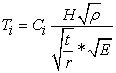(1)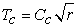(2)

Which above parameters are as follow:

t: equivalent thickness of tank wall

p: weight volume of the fluid

r: diameter of the tank

E: module of elasticity

H: The height of the fluid in the tank

Cc and Ci: Non-dimension coefficients

For reservoirs with variable wall thickness, equivalent thickness can be calculated based on weighted average method.

• Calculating the equivalent thickness for  tank with the proportion of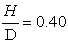using weight method: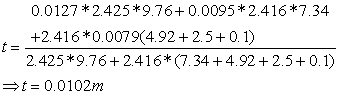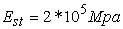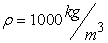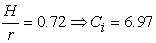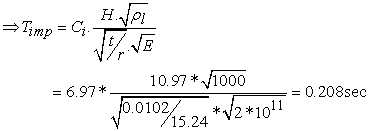(3)

After doing the analyses, the summary of results is as follow:

#### Table 6. Comparison of dominant periodic time of current study with ‎[19,20]

In this study, in order to calculate the natural periods and shape-modes of fluid-tank system, frequency range of 1 to 7 Hz is considered. Modal analysis was used to determine the natural frequency and its shape-modes in the mentioned frequency. In order to extract and calculate the modes and eigenvalues Block, Lanczos and Reduced methods were used which in the following the results of Modal analysis is presented.

### 5. Results of Modal Analysis

Usually for most of the structures which are exposed to vibration, running the Modal analysis is essential, because the structure should be designed to be far from the resonant frequency domain. Also this analysis is used to determine natural frequencies and seismic mode-shapes of the structure. The value of natural frequency of the structure depends on the shape, type and supports of the structure. For cylindrical tanks containing fluids, this is important that modes of the structure occur at high frequencies and modes of the fluid occur at low frequencies. Modes related to the fluid which are famous as oscillation modes, appear at pristine frequencies of Modal analysis. However all or some of the pristine modes may not be important, and is a result of internal rotational motion in the fluid. Structural modes or pulse modes as the stiffness of the tank wall may occur at higher modes. Important modes at the vibration of structure are determined according to the factors such as participation ratio, Modal coefficient and percent of distribution mass. In Figure 24 to Figure 29 instances of vibration modes of fluid-tank system in the proper state is shown after Modal analysis for first 7 modes.

Figure 30 to Figure 36show the variation of mode-shapes of proper tank after doing the Modal analysis

### 6. Obtained Results from Modal Analysis

In this study Modal analysis was done to consider the accuracy of tank modeling and obtaining the natural frequency of the tank. In Table 7, first natural frequency of first tank is presented.

#### Table 7. Obtained frequency of Modal analysis of proper tank

Also Figure 38 and Figure 37 shows the changesin the figure.

### 7. Results of Harmonic Analysis

Usually this analysis is done after the modal analysis. This analysis approximately presents the natural frequencies of the system. In this analysis, system is under a horizontal acceleration equals to 0.3g exerted to the base of the tank which is the design basis acceleration suggested by Iranian Seismic Code for structures located on the relative high danger areas. In the analyses, the overall damping matrix of the system is the summation of damping matrixes of fluid viscose elements and Riley matrix which is a function of mass and stiffness matrixes and is as follow:

That [CF] is the damping matrix of viscose fluid element. In order to obtain the damping matrix of the fluid, stress and strain derivative relation which basically links the time changes of strain by viscosity coefficient to shear stress is used. In order to perform dynamic damping analyses, we need to calculate the constant coefficients α and β which are achieved with simplification as the following relations (reference of failure mode consideration).

To evaluate these coefficients, the damping ratio of the system is assumed in the range of 3 to 4 percent as it is shown in Figure 39. By solving the above equation, we will reach a simpler relation to evaluate the Riley damping coefficients. In the above relation Wi and Wj are the angular frequencies of two main modes including oscillation mode of fluid and pulse mode of structure. In addition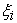and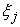are the corresponding damping ratios ‎.

By considering the mentioned frequency range and presented formulas, α and β are 0.80 and 0.0012 respectively. Figure 40 to Figure 46show the instances of vibrating modes of fluid-tank after performing Harmonic analysis for some pristine modes.

Figure 47 to Figure 53 show the changes of 7 pristine modes of the tank.

### 8. Calculation of Governing Mode Period Based on the Presented Relations by Veletsos et al

Dynamic analysis of presented model is developed based on the idea of the systems with the one degree of freedom.

Relations by Veletsos to calculate the period time of impulsive and convective mode was introduced in the previous sections. In the following the method of evaluating the governing periodic mode of the tank using Veletsos relation is presented and is compared with the results of Modal analysis.

1. First Mode (Proper Tank):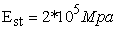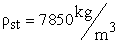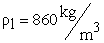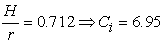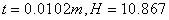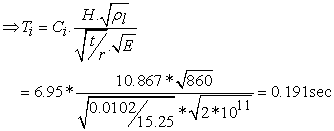(4)

2. Second Mode (Tank Susceptible to Corrosion)

The reduction of thickness after five years: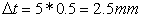remained thickness of the tank wall: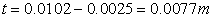Calculation of equivalent thickness: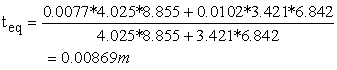In Figure 54, the geometric dimensions of the tank wall after corrosion is shown.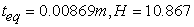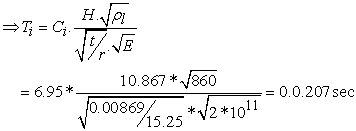(5)

Results obtained from the Modal analysis and also the periods obtained from the Veletsosrelation are shown in Table 8. As it can be seen, results from the current study are compared with the theoretical results and finally the amount of error available in the results is introduced as percentage.

#### Table 8. Comparison of obtained period time from Modal analysis and Veletsos relation

According to the results from the Modal analysis which is presented in the above Table and its comparison with results of available models, the modeling procedure and analysis steps are verified suitably.

Usually the conformity of resonant frequency with frequency of the main modes of the structure indicates the accuracy of Modal analysis which the following Table briefly shows the results of Modal and Harmonic analysis that demonstrates the accuracy of modeling steps and analysis in the software.

### 9. Mass Equivalent Method

This method was first presented by Ranjbaran et al (2010) to consider the dynamic features of cracked bars using the mass equivalent. In this method, first they removed the cracks of a designed cantilever beamand then they substituted the cracked structure with an equivalent mass which is able to equal the influences of the former crack. Because modeling the defects such as surface cracks and holes in the thin wall structures by finite element software is a hard and time consuming work, thus it was determined to use this method to design corrosion of the tanks. In this study according to the obtained frequencies from the cracked tank, a proper tank was designed and in the place of the corrosion, a mass (from the same type of the tank wall) was added. It is notable that the added mass should have no connection to the internal wall of the tank. Meanwhile as it is shown in Figure 57, using this method in the analysis steps, the place of the tank supports will change.

Because in the previous step, the reduction in the thickness of the tank wall resulted in the reduction of the stiffness of the structure and hence the reduction of the frequency of the system, so in the second step, by adding the mass, the reverse action occurred. It means by adding some mass, the denominator of the frequency increases which results in the reduction of the frequency. This increasing mass will be repeated until the frequency of the system in the corrosion step will equal to the frequency which is obtained from this step. The mass which from it the frequency is achieved is called the equivalent mass, which in this study, the equivalent mass is obtained by repeating the Transient analysis in several steps.

### 10. Results of Transient Analysis

In the transient analysis step, according to the objective of this research which is the equivalent mass to consider the corrosion phenomenon on the dynamic features of reservoirs, as it was mentioned previously, one model of cylindrical tank along with corrosion exerted to the internal wall of it was designed in the software and was exposed to the horizontal acceleration of earthquake. In order to gain an equivalent frequency equal to the frequency of the tank which is susceptible to corrosion, three steps of increased mass to the tank was done (models 1 to 3 respectively). The height of additional masses is equal to the height of the corrosion but their thickness is variable (0.0102m, 0.008m and 0.005m). In Figure 58 to Figure 60, the geometry of three models with the added mass is presented after modeling and software analysis.

Also the frequency of governing mode obtained from the transient analysis for all three modes is presented in the following Table. Figures of corresponding modes with frequencies is shown in the following as well.

#### Table 10. Comparison of period time obtained from the Modal and Harmonic analysis for all three models with the added mass

Changes in mode-shapes formed in the fluid-tank system in all three models are shown in Figure 61 to Figure 63 after the analysis.

After doing these steps, by using the equivalent mass and transient analysis methods, frequencies of system and changes of tank features should be compared. As it can be seen from Figure 64 to Figure 66, the peak points of these figures represent the resonance phenomenon and periodic time of governing mode.

Here after the software analysis on the models of tanks which are susceptible and three models of tanks with added mass, the change in stress in proportion to height is shown as graphs in the following.

### 13. Comparison of Displacement Graphs in Proportion to Stimulation Period

Figure 77. Comparison of displacement changes in the axial direction in proportion to periodic stimulation of tank susceptible to corrosion or model 1
Figure 78. Comparison of displacement changes in the axial direction in proportion to periodic stimulation of tank susceptible to corrosion or model 2
Figure 79. Comparison of displacement changes in the axial direction in proportion to periodic stimulation of tank susceptible to corrosion or model 3

In the following, the final result of objective frequency and frequency of the tank susceptible to corrosion are considered.

#### Table 12. Comparison of periodic time obtained from the Harmonic analysis with models 1, 2 and 3

After the analyses by the software and drawing the graphs of mode deformations and stress changes on the wall of the tanks, and also the displacement in axial direction, some graphs are presented in order to consider and compare three designed models. In addition, in the above table, corresponding frequency to each model is compared with the tank which is susceptible to corrosion. It is notable that as it can be understood from the figures and analyses, graphs of model 3 which represents the proper tank containing added mass of 0.005m thickness has the lowest difference and model 1 has the highest difference in comparison with the graphs and frequencies of the tank susceptible to corrosion. In the other words, a mass with the same height of corrosion and 0.005m thickness in the place of corrosion can satisfy the corrosion conditions in the cylindrical steel tank under the bases forces and in order to model and analyze these tanks, we can use this alternative model.

### 14. Conclusion and Suggestions

14.1. Conclusion

After doing the analyses, considerations and comparison of results on the models, in this section we summarized and concluded this study. Additionally some suggestions are presented for further researches in the future.

The main aim of this study is to introduce an equivalent mass method to consider the corrosion phenomenon on the dynamic properties of the reservoirs. In this research, in the first step the finite element method was used to model two steel cylindrical tanks containing the specific fluid with the proportion of diameter to height equals to 0.4. In the first model, a cylindrical tank with specific dimensions was modeled as a proper tank. In the second model, that tank was exposed to some corrosion on the internal wall of the tank while the amount of the fluid was constant. In order to consider the corrosion and thickness reduction on the wall of the tank, in accordance with References ‎[17, 20], the height of the tank was divided into three zones. The value of thickness reduction on the wall in two zones of lower belt which had the most destructive effects was the average of 0.5 mm yearly. Also the thickness reduction in the lower part of the tank is negligible. According to the previous studies about the relation of age of the tank and the amount of corrosion during the utilization, the useful life of tanks was found to be usually between 20 to 40 years which in most of the cases, after 1.5 to 2.5 years, corrosion occurs. As a conclusion, according to the initial thickness of the tank, thickness reduction was exerted after 5 years of the reservoirs’ lives.

In order to consider the influences of corrosion in the geometry of model, finite element software (ANSYS) was used for modeling and analysis. Shell as a finite element model was used for the floor and roof elements. Also in order to model the fluid inside the tank, fluid element was used. Mentioned elements were used to model an experimental model ‎ and finally appropriate results were obtained from it. It is remarkable that one of the most important steps in modeling is to apply the boundary conditions to the system.

Fluid elements in the common border can only move in the direction perpendicular to the wall of the tanks which means x and y axes. Therefore in the modeling procedure, the common nodes of fluid and structure were coupled to each other in the perpendicular direction to the walls of the tank. Based on the threatening danger of earthquake for most regions of our country, the design bases acceleration was assumed to be 0.3g. After doing the Modal analysis and gaining the natural frequencies of the tank, control of the Harmonic analysis was done by exerting a basic stimulating force on the model susceptible to corrosion. This analysis which is done usually after the Modal analysis, presents the natural frequencies of the system approximately. Then in order to apply the overall matrix of the system, the summation of damping matrix elements and Riley matrix were used. Results of these analyses are presented in the separate tables and figures. Finally by using the equivalent mass method, some models of the tank were modeled using the thicknesses of 0.0102m, 0.008m and 0.005m and after applying the boundary conditions and loading, their frequency was obtained by doing the transient analysis for three models. For the equivalent mass method, according to the obtained frequencies of the tank susceptible to corrosion, one model of the proper tank along with a layer of added mass from the same type of the tank, was added to the place of corrosion and was modeled. This adding the mass continued until the frequencies of the governing mode from this analysis equal to the frequencies of the tank susceptible to corrosion.

After running the analyses by the ANSYS software and considering it on the designed models, the following results are gained:

1. Occurrence of corrosion in the wall of the tank reduces the stiffness of the system which it causes to increase the formed modes in a limited range of frequencies.

2. The change in stress in the upper belt of the tank, with the proportion of height to diameter equals to 0.4 is almost constant, while the distribution of pressure in the middle belt had the highest changes with the passing time.

3. In the equivalent mass, the exerted mass has a scalar state and affects on the influence point of it while the modeling of thickness changes in one point is difficult and bothersome. This method is a simple way for modeling. Meanwhile the accuracy of computations in this method is truly high and also the amount of calculations has reduced.

4. As the thickness of the mass (added to the wall of the tank) reduces to some levels, the periodic time from analyses approaches the periodic time of the tank susceptible to corrosion and after that level, it increases.

5. As it can be seen from the figures, the place of confluence for the figures of corrosion tank and models 1,2 and 3 is in the lower area of the tank. As we move far from the bottom of the tank, this distance increases. It means the maximum changes in displacement of mode-shapes of the tanks in all three states, even by containing the added mass, is still in the upper area of the tank.

6. The results from the Modal analysis showed that the surface of the fluid, in its maximum displacement, however it has short distance from the roof, encounter to it and the roof of the tank was influence even when the distance was assumed to be a little more than the suggestion of the Code.

7. ANSYS software has various capabilities to analyze the structures and interaction of the fluid-structure. We should notice that this product can do the Modal analysis linearly and we should neglect any non-linear property in the definitions.

### References

  Cho. J. R, Song. J. M, Lee. J. K “ Finite element techniques for the free-vibration and seismic analysis of liquid-storage tanks” Finite Elements in Analysis and Design, Vol 37, 2001, pp 467-483.In article CrossRef  Shrimali. M. K, Jangid. R.S “ Seismic response of liquid storage tanks isolated by sliding bearings” Engineering Structures, Vol 24, 2002, pp 909-921.In article CrossRef  Fan Jiashen, Li Jibin “ Dynamic stability of liquid storage cylindrical tanks subjected to horizontal seismic force excitation” Applied Mathematical Modelling, Vol 18, 1994, pp 373-383.In article CrossRef  Shrimali. M. K, Jangid. R. S “Non-linear seismic response of base-isolated liquid storage tanks to bi-directional excitation” Nuclear Engineering and Design, Vol 217, 2002, pp 1-20.In article CrossRef  Kyung Hwan Cho, Moon Kyum Kim, Yun Mook Lim, Seong Yong Cho “Seismic response of base-isolated liquid storage tanks considering fluid–structure–soil interaction in time domain” Soil Dynamics and Earthquake Engineering, Vol 24, 2004, pp 839-852.In article CrossRef  Pranesh Chatterjee, Biswajit Basu. “Nonstationary seismic response of a tank on a bilinear hysteretic soil using wavelet transform” Probabilistic Engineering Mechanics, Vol 21, 2006, pp 54-63.In article CrossRef  Hugo Hernández-Barrios, Ernesto Heredia-Zavoni, Álvaro A. Aldama-Rodríguez “Nonlinear sloshing response of cylindrical tanks subjected to earthquake ground motion” Engineering Structures, Vol 29, 2007, pp 3364-3376.In article CrossRef  Panchal. V. R, Jangid. R. S “Variable friction pendulum system for seismic isolation of liquid storage tanks” Nuclear Engineering and Design, Vol 238, 2008, pp 1304-1315.In article CrossRef  Ahari. Nourali, Eshghi. Masoud, Ghafory. Sassan, Ashtiany. Mohsen “The tapered beam model for bottom plate uplift analysis of unanchored cylindrical steel storage tanks” Engineering Structures, Vol 312009, pp 623-632.In article  Dehghan Manshadi. S. H, Maheri. Mahmoud. R “The effects of long-term corrosion on the dynamic characteristics of ground based cylindrical liquid storage tanks” Thin-Walled Structures, Vol 48, 2010, pp 888-896.In article CrossRef  Ayman. A. Seleemah, Mohamed. El-Sharkawy “Seismic response of base isolated liquid storage ground tanks” Ain Shams Engineering Journal, Vol 2, 2011, pp 33-42.In article CrossRef  Moslemi. M, Kianoush. M. R “Parametric study on dynamic behavior of cylindrical ground-supported tank“Engineering Structures, Vol 42, 2012, pp 214-230.In article CrossRef  A. Zagorski, H. Matysiak, O. Tsyrulnyk, O. Zvirko, H. Nykyforchyn, and K. Kurzydlowski. “Corrosion and stress-corrosion cracking of exploited storage tank steel”. Materials Science, Vol. 40, No. 3, 2004.In article  Veletsos AS,Yang JY. “Earthquake response of liquid storage tanks advences in civil engineering through mechanics”. Proceedings of the second engineering mechanics specialty conference.Raleigh(NC):ASCE,1977.pp 1-24.In article  Haroun, M.A., 1983, “Vibration studies and tests of liquid storage tanks”,Earthquake Engineering&Structural Dynamics, 2, 179-206.In article CrossRef  Maheri, M.R., Severn, R.T., (1986), “Hydrodynamic effects in steel liquid storage tanks”, Steel structures, Recent Research Advances and their Applications to Design, 483-508.In article  Medvedea, M.L., and Tiam, T.D., (1998), “Classification of corrosion damage in steel storage tanks”, chemical and petroleum engineering, 9-10.In article  Ranjbaran, A., Shokrzadeh, A.R., Khosravi. S., 2010, “A new finite element analysis of free axial vibration of cracked bars”, International Jornal For Numerical Methods inBiomedical Engineering.In article  Veletsos, A.S., Yang, J.Y., 1977, “earthquake response of liquid storage tanks advences in civil engineering through mechanics”, Proceedings of the second engineering mechanics specialty conference, 1-24.In article  Virella, J.C., Godoy, L.A., Su´arez, L.A., 2003, “Fundamental modes of tank-liquid systems under horizontal motions”, Engineering Structures, 28, 1450-1461.In article CrossRef